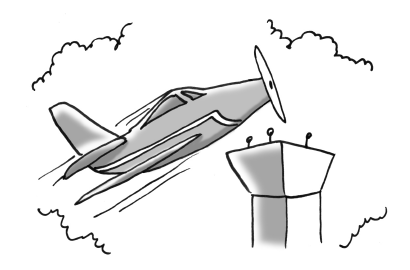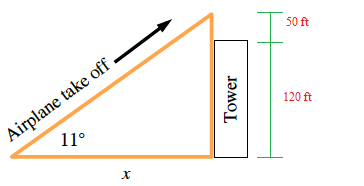### Home > INT2 > Chapter 4 > Lesson 4.2.4 > Problem4-94

4-94.An airplane takes off and climbs at an angle of $11^\circ$. If the plane must fly over a $120$-foot tower with at least $50$ feet of clearance, what is the minimum distance between the point where the plane leaves the ground and the base of the tower?

1. Draw and label a diagram for this situation.

2. What is the minimum distance between the point where the plane leaves the ground and the tower? Explain completely

How can you use a slope ratio to find the minimum distance?

The slope ratio for $11^\circ$ is $\frac{1}{5}$.